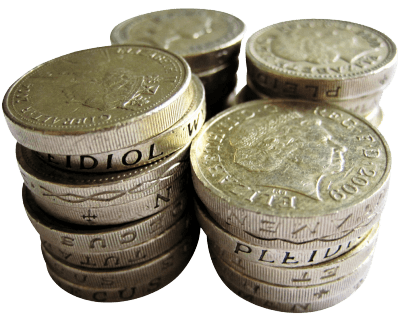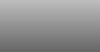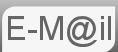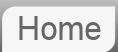# Answer to Puzzle #43: 10 Piles of 10 Coins one of Which is Fake.

43. You have 10 stacks of 10 gold coins. All of the coins in one of these stacks are counterfeit, all the other coins are not. A real coin weighs 10 grammes. A counterfeit coin weighs 11 grammes. You have a modern scale that provides an accurate readout. What is the minimum number of weighings needed to determine which stack is fake?

Another 'weighings' riddle right? I know many of you will have heard this before, and of course if you have it's very easy; though there are still some points I would like to make. If you haven't it's an impressive deduction if you can work it out. Note this is a modern scale, not an old-fashioned balance.Before reading the answer can I interest you in a clue?

So straight in to the answer we number the piles 1 through 10. We take one coin from pile #1, two coins from pile #2, three coins from pile #3 and so on until we have 10 coins from pile #10.

This gives us 55 coins which if they were all pure would give us 550g. However, some of them will be fake. Lets say we place them all on the scale, for our one and only weighing, and it reads 553g. The only possible way this could have happened is if we placed 3 counterfeit coins on the balance, and that means that pile #3 is counterfeit.

## 11 Stacks of 10 Coins

In every practical sense you do the same thing as before. We just consider the additional pile as pile #0. Then the rule we established earlier still holds that the pile number is given by the actual weight minus the expected weight. The only difference now is that we allow for the possibility of the expected weight being equal to the observed weight, which points to pile #0.

There is a lesson here about the importance of zero.

## Why is this important?

This technique is actually used, in real life, in a number of areas. What follows is a very simplified version of Reed Solomon Error Correction as used in, for example Audio CDs. Ignore this if you like, it's nothing to do with the puzzle.

A CD is essentially a series of numbers stored on the disk. The problem is when you come to read those numbers back you may get something else. You need to be able to verify the data is correct and if not identify and correct the fault with the minimum amount of extra data having to be included. Suppose we wish to store the following sequence of numbers:

5 11 -3 6 0 -9 8 -2

The additional data we will calculate is the sum of all the numbers, in this case 16 and the sum of the numbers times their position. As in multiply each number by it's position and then sum.

0*5 + 1*11 + 2*(-3) + 3*6 + 4*0 +5*(-9) + 6*8 + 7*(-2) = 0 + 11 - 6 + 18 + 0 - 45 + 48 - 14 = 12

We will store these two numbers, the sum and position sum together with our original data. Like this 5 11 -3 6 0 -9 8 -2, 16, 12. Now suppose when we read the data back it has been slightly corrupted and looks like this:

5 11 -3 6 0 -9 5 -2, 16, 12

We recalculate the sum and the position sum the same way as before to give 13 and -6 respectively. Comparing the sums 16(correct) against 13(calculated) we can see the magnitude of the error is -3. We know one of the numbers is 3 too low.

The original 'position sum' was 12 the new one calculates to -6 meaning our position sum has changed by -18. Knowing as we do, that the magnitude of the error was -3 we can divide -18 by -3 to get the position, 6, the 6th number is 3 too low. Change that 5 to an 8 and we have restored our original sequence.

Hopefully you can see that what we did with the coins was a simplified version of this. This in fact is the whole reason I included this puzzle.

© Nigel Coldwell 2004 -  – The questions on this site may be reproduced without further permission, I do not claim copyright over them. The answers are mine and may not be reproduced without my expressed prior consent. Please inquire using the link at the top of the page. Secure version of this page.

PayPal
I always think it's arrogant to add a donate button, but it has been requested. If I help you get a job though, you could buy me a pint! - nigel